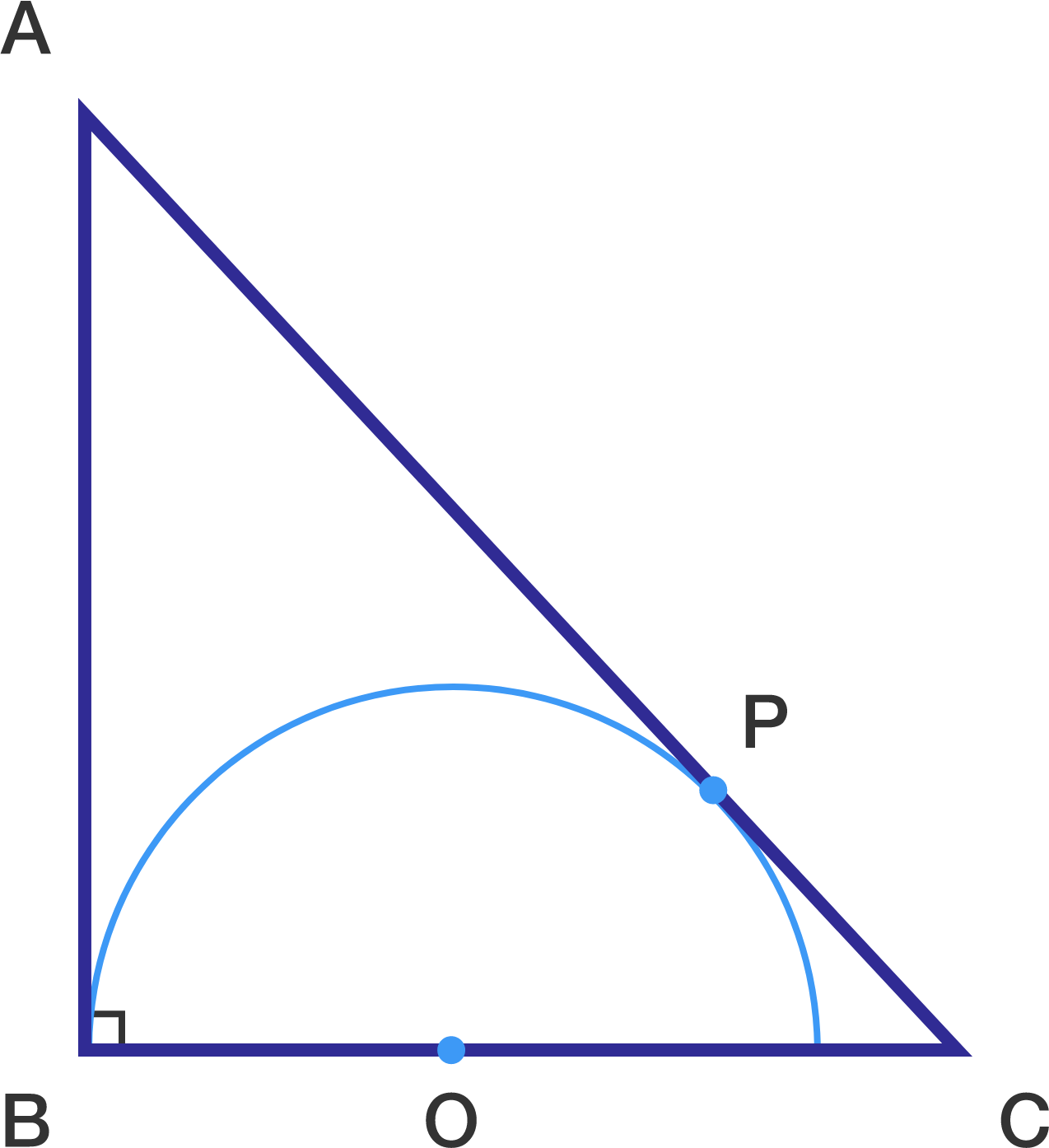# Semi-circle is all set to blow your mind !

Geometry Level 2Given a right triangle $\Delta ABC$ ; right angled at B .

If the measure of $AB$ , $BC$ and $CA$ are $3$ , $4$ and $5$ units respectively .

If the radius i.e $\overline{OB}$ of the circle with centre $O$ as shown , can be represented as $\dfrac{a}{b}$ , where $a$ and $b$ are coprime .

Find $a + b$.

×